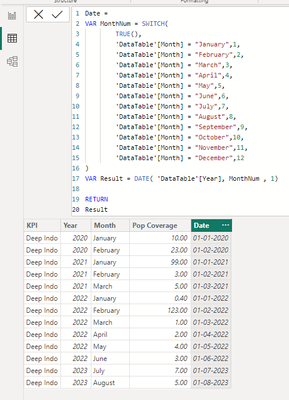cancel
Showing results for
Did you mean:Frequent Visitor

## Table Repeat value based on other column

Hi All,

I want to create a calculation "Baseline" like the one below that contains the 1st value from [Pop Coverage] col and its repeated though the whole [Year] Col.1 ACCEPTED SOLUTIONSuper User

Here is my solution:

1. Create a calculate column for Date in the table using the below DAX:

`Date = VAR MonthNum = SWITCH( TRUE(),'DataTable'[Month] = "January",1,'DataTable'[Month] = "February",2,'DataTable'[Month] = "March",3,'DataTable'[Month] = "April",4,'DataTable'[Month] = "May",5,'DataTable'[Month] = "June",6,'DataTable'[Month] = "July",7,'DataTable'[Month] = "August",8,'DataTable'[Month] = "September",9,'DataTable'[Month] = "October",10,'DataTable'[Month] = "November",11,'DataTable'[Month] = "December",12)VAR Result = DATE( 'DataTable'[Year], MonthNum , 1)RETURNResult`

Table Snapshot:`2. Create a measure for Baseline:Baseline =VAR MinDate =CALCULATE (MIN ( 'DataTable'[Date] ),ALL ( 'DataTable' ),'DataTable'[Year] = SELECTEDVALUE ( 'DataTable'[Year] ))VAR Result =CALCULATE (MIN ( 'DataTable'[Pop Coverage] ),ALL ( 'DataTable' ),'DataTable'[Year] = SELECTEDVALUE ( 'DataTable'[Year] ),'DataTable'[Date] = MinDate)RETURNResult`

Output Snapshot:Hope this helps.

 ** If you liked my solution, please give it a thumbs up. And if I did answer your question, please mark this post as a solution, so that it comes in top of the search and help others. Thank you ! Good Luck 👍

3 REPLIES 3Super User

Here is my solution:

1. Create a calculate column for Date in the table using the below DAX:

`Date = VAR MonthNum = SWITCH( TRUE(),'DataTable'[Month] = "January",1,'DataTable'[Month] = "February",2,'DataTable'[Month] = "March",3,'DataTable'[Month] = "April",4,'DataTable'[Month] = "May",5,'DataTable'[Month] = "June",6,'DataTable'[Month] = "July",7,'DataTable'[Month] = "August",8,'DataTable'[Month] = "September",9,'DataTable'[Month] = "October",10,'DataTable'[Month] = "November",11,'DataTable'[Month] = "December",12)VAR Result = DATE( 'DataTable'[Year], MonthNum , 1)RETURNResult`

Table Snapshot:`2. Create a measure for Baseline:Baseline =VAR MinDate =CALCULATE (MIN ( 'DataTable'[Date] ),ALL ( 'DataTable' ),'DataTable'[Year] = SELECTEDVALUE ( 'DataTable'[Year] ))VAR Result =CALCULATE (MIN ( 'DataTable'[Pop Coverage] ),ALL ( 'DataTable' ),'DataTable'[Year] = SELECTEDVALUE ( 'DataTable'[Year] ),'DataTable'[Date] = MinDate)RETURNResult`

Output Snapshot:Hope this helps.

 ** If you liked my solution, please give it a thumbs up. And if I did answer your question, please mark this post as a solution, so that it comes in top of the search and help others. Thank you ! Good Luck 👍Frequent Visitor

Thank you!!! 🙂 it worksSuper User

@PatCreator You are welcome 😀👍